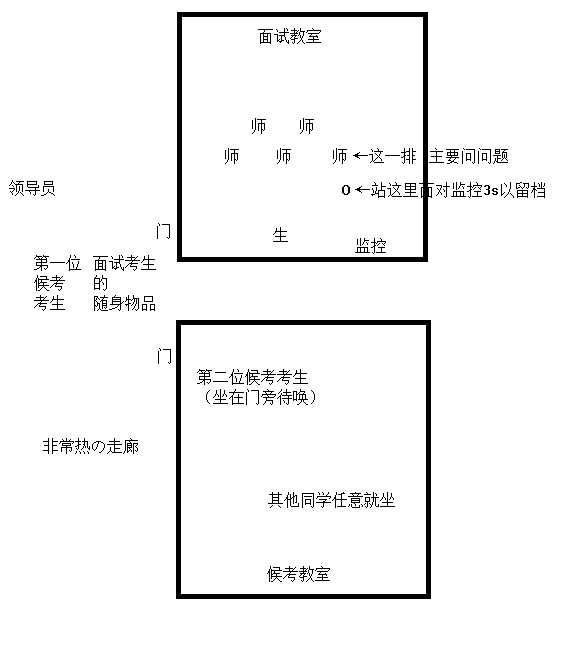# 初赛

1. (3pts) X,Y\in [0,1],在XY随机取的情况下，满足X^2+Y^2\leq1且(X-1)^2+(Y-1)^2\leq1的概率是

2. (3pts) \frac{1}{x}+\frac{1}{y}=\frac{1}{30}(x\leq y)的正整数解个数

3. (3pts)人以v的水平速度从倾角为\theta的斜坡上无阻力飞出，离斜面距离最远是多少。重力加速度已知为g。

4. (5pts)一个正整数在3进制下的数位和记为a_n，例如a_9=1,a_7=3，求|\{x|a_x=5,1\leq x\leq 2023\}|

5. (5pts)abc常数，x+y=c,\sqrt{x^2+a^2}+\sqrt{y^2+b^2}最小值?

6. (5pts)在一辆做半径为r，速度为v水平向左的圆周运动车中有一个水杯，水杯下有向右的小孔在漏水，水的高度是h，问：车中的观察者观察到的水从小孔中射出的速度？

7. (3pts)有一个正方形ABCD在与该正方形所在平面平行的匀强电场中，B点电势比A点高\sqrt6，比C点高\sqrt2，求电场强度，CD电势差

8. (5pts)求\sum_{k=1}^n \gcd(k^2+2k+3,n)，其中n=100

# 复赛

1. 在三角形ABC中，a、b、c都是有理数，则\cos(nA)一定是有理数吗？（其中n=1,2,3,4...）sinA一定是有理数吗？

2. 在X轴上有一质点在原点，每一时刻有p概率向右移动一个单位，(1-p)时刻向左移动一个单位，问：n时刻停在原点的概率？

3. 一个（补充：绝热）气缸，中间有隔板挡开，左侧是气体，右侧是真空，抽开隔板，气体的内能改变吗、如何改变？THE END